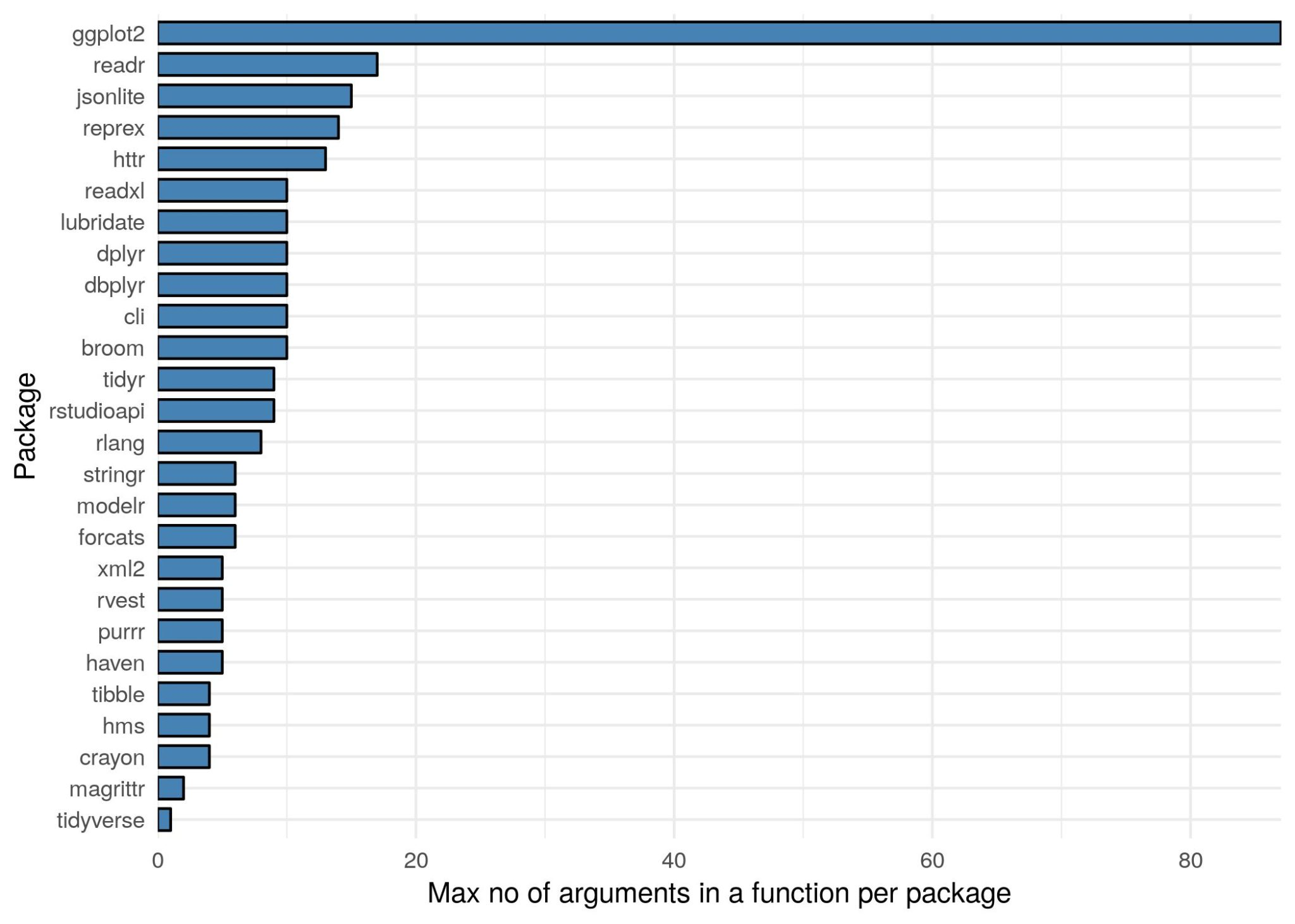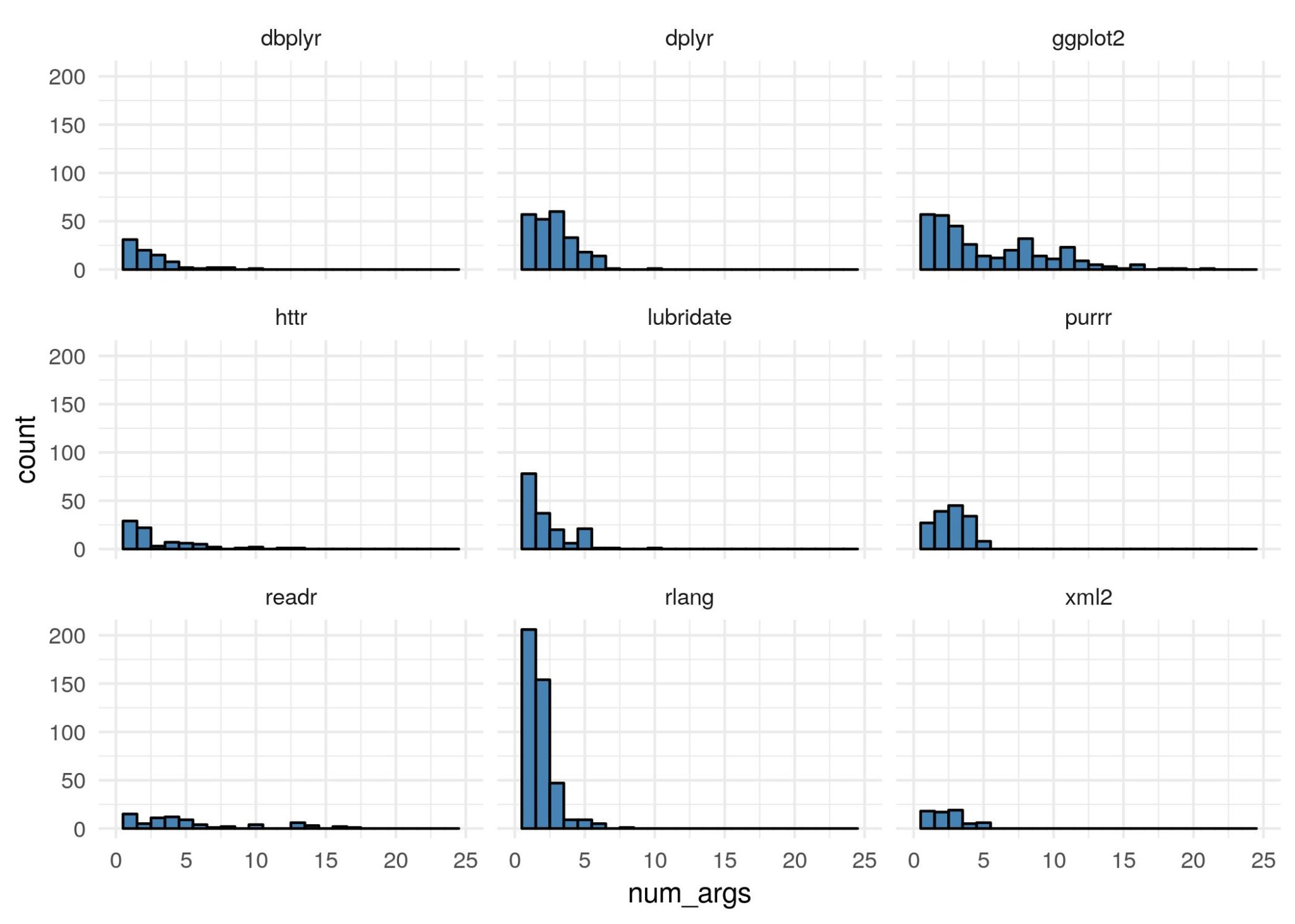Want to share your content on R-bloggers? click here if you have a blog, or here if you don't.

Before we start anything, I’d like to mention that most of the hard work came from nsaunders and his great blog post Idle thoughts lead to R internals: how to count function arguments.

Let’s get started.

The aim of this blog is to capture the number of arguments present in each function with packages of the tidyverse. First we need to load the necessary packages

```library("tidyverse")
library("tidytext")```

Now we need to grab the relevant tidyverse packages

```tpkg = tidyverse_packages()

##  "broom"   "cli"     "crayon"  "dplyr"   "dbplyr"  "forcats"```

We’ve had to reset the 17th element to readxl as it gets loaded as `readxl\n(>=`, which breaks the next block of code. Now we also need to load in the tidyverse packages. Doing this one by one would be a pain, so I’ve used `map()`

`map(tpkg, library, character.only = TRUE)`

Now for the actual analysis I’m just going to whack the full code in now, then go through it line by line.

```pkg = tpkg %>%
as_tibble() %>%
rename(package = value) %>%
rowwise() %>%
mutate(funcs = paste0(ls(paste0("package:", package)), collapse = ",")) %>%
unnest_tokens(func, funcs, token = stringr::str_split,
pattern = ",", to_lower = FALSE) %>%
filter(is.function(get(func, pos = paste0("package:", package)))) %>%
mutate(num_args = length(formalArgs(args(get(func, pos = paste0("package:", package)))))) %>%
ungroup()```

This is what the head of `pkg` looks like

```head(pkg)

## # A tibble: 6 x 3
##   package func            num_args
##   <chr>   <chr>              <int>
## 1 broom   augment                2
## 2 broom   augment_columns        8
## 3 broom   bootstrap              3
## 4 broom   confint_tidy           4
## 5 broom   finish_glance          2
## 6 broom   fix_data_frame         3```

### Lines `1-4`

Lines 1-4 look like this

```tpkg %>%
as_tibble() %>%
rename(package = value) %>%
rowwise() %>%```

Here we are grabbing, the tidyverse packages character vector, converting it to a tibble and renaming the column. We then use `rowwise()` so that we can work in a row-wise fashion.

### Line 5

`mutate(funcs = paste0(ls(paste0("package:", package)), collapse = ",")) %>%`

To get a character vector back of the objects within a package, we do `ls("package:package_name")`. However, we want to store this as a single string, so we need to use our old friend `paste0()` to do so. We then use mutate to attach this to the data frame. Our data from now looks like this

```## Source: local data frame [6 x 2]
## Groups: <by row>
##
## # A tibble: 6 x 2
##   package funcs
##   <chr>   <chr>
## 1 broom   argument_glossary,augment,augment_columns,bootstrap,column_gloss…
## 2 cli     ansi_hide_cursor,ansi_show_cursor,ansi_with_hidden_cursor,bg_bla…
## 3 crayon  %+%,bgBlack,bgBlue,bgCyan,bgGreen,bgMagenta,bgRed,bgWhite,bgYell…
## 6 forcats %>%,as_factor,fct_anon,fct_c,fct_collapse,fct_count,fct_cross,fc…```

### Lines 6 – 7

```unnest_tokens(func, funcs, token = stringr::str_split,
pattern = ",", to_lower = FALSE) %>%```

As we’ve stored the function names as a single string, we can now apply some tidytext to turn our data into long data! We do this using the `unnest_tokens()` function. Here we are taking the `funcs` variable, turning it into `func` by splitting it up using `str_split()` from stringr. The data now looks like this

```## Source: local data frame [6 x 2]
## Groups: <by row>
##
## # A tibble: 6 x 2
##   package func
##   <chr>   <chr>
## 1 broom   argument_glossary
## 2 broom   augment
## 3 broom   augment_columns
## 4 broom   bootstrap
## 5 broom   column_glossary
## 6 broom   confint_tidy```

### Line 8

`filter(is.function(get(func, pos = paste0("package:", package)))) %>%`

Now, not every object inside a package is a function. We can use `is.function()` to test this. However, as our function names are stored as strings, we must wrap them in the `get()` function. For instance,

```is.function("augment")

##  FALSE

is.function(get("augment"))

##  TRUE```

What if we have conflicts in function names? We can also specify the package our function is from, using the argument `pos`

```is.function(get("augment", pos = "package:broom"))

##  TRUE```

We can then use this condition within a filter command to remove any objects that aren’t functions

### Lines 9 – end

```mutate(num_args = length(formalArgs(args(get(func, pos = paste0("package:", package)))))) %>%
ungroup()```

It is possible to withdraw the arguments of a function using the `formalArgs()` function. However, this does not work on primitive functions

```formalArgs(get("add", pos = "package:magrittr"))

## NULL

formalArgs(get("augment", pos = "package:broom"))

##  "x"   "..."```

We can counter act this by wrapping the function in `args()` first. This method now works for both primitives and non-primitives

```formalArgs(args(get("add", pos = "package:magrittr")))

##  "e1" "e2"

formalArgs(args(get("augment", pos = "package:broom")))

##  "x"   "..."```

To work out the number of these argument we simply wrap this expression in `length()`.

### The big reveal

```pkg %>%
arrange(desc(num_args))

## # A tibble: 2,292 x 3
##    package    func               num_args
##    <chr>      <chr>                 <int>
##  1 ggplot2    theme                    93
##  2 ggplot2    guide_colorbar           28
##  3 ggplot2    guide_colourbar          28
##  4 ggplot2    guide_legend             21
##  5 rstudioapi launcherSubmitJob        21
##  6 ggplot2    geom_dotplot             19
##  7 ggplot2    geom_boxplot             18
## # … with 2,282 more rows```

So it turns out that `theme()` from ggplot2 is king of the arguments, by a mile! The largest per package looks like thisWe’re not done there! The 9 packages with the largest sum of arguments are

```largest = pkg %>%
group_by(package) %>%
count() %>%
arrange(desc(n)) %>%
pull(package)
largest

##  "rlang"      "ggplot2"    "dplyr"      "purrr"      "lubridate"
##  "dbplyr"     "readr"      "rstudioapi" "httr"```

We can plot a histogram, for each package, of the no. of arguments in each function like so..

```pkg %>%
filter(package %in% largest) %>%
ggplot(aes(x = num_args)) +
geom_histogram(binwidth = 1, fill = "steelblue", col = "black") +
facet_wrap(~package) +
xlim(c(0, 25)) +
theme_minimal()```We can go a step further and retrieve the argument names as well. To do this we use the same technique as before with the functions

```pkg %>%
rowwise() %>%
mutate(args = paste0(formalArgs(args(get(func, pos = paste0("package:", package)))),
collapse = ",")) %>%
unnest_tokens(arg, args, token = stringr::str_split,
pattern = ",", to_lower = FALSE) %>%
ungroup() %>%
count(arg) %>%
arrange(desc(n))

## # A tibble: 1,029 x 2
##    arg          n
##    <chr>    <int>
##  1 ...        785
##  2 x          698
##  3 data       169
##  4 .x         120
##  5 ""         102
##  6 n           91
##  7 .f          90
##  8 position    90
##  9 mapping     79
## 10 na.rm       79
## # … with 1,019 more rows```

The most commonly used arguments in the tidyverse are `...` and `x` by some distance.

```pkg %>%
rowwise() %>%
mutate(args = paste0(formalArgs(args(get(func, pos = paste0("package:", package)))),
collapse = ",")) %>%
unnest_tokens(arg, args, token = stringr::str_split,
pattern = ",", to_lower = FALSE) %>%
ungroup() %>%
group_by(package) %>%
count(arg) %>%
arrange(package, desc(n)) %>%
slice(2) %>%
arrange(desc(n))

## # A tibble: 26 x 3
## # Groups:   package 
##    package   arg         n
##    <chr>     <chr>   <int>
##  1 ggplot2   data      103
##  2 purrr     .x         91
##  3 dplyr     x          83
##  4 rlang     ...        64
##  6 lubridate ...        35
##  7 stringr   pattern    23
##  8 dbplyr    x          21
##  9 httr      ...        21
## 10 tidyr     ...        18
## # … with 16 more rows```

So you can see that `data` is the most common argument within ggplot2, `.x` is the most common argument within purrr and so on…

That’s it for this blog post. Hope you’ve enjoyed!The post Counting Arguments in the Tidyverse appeared first on Jumping Rivers.